# How to Skip First Item in the Array in PowerShell

## Problem#

When we create PowerShell script, there might be a case when we want to export an object to CSV file and we don’t want to include the first item which is the header or column names, so we have to skip it.

In this blog post, we will show you how to skip the first array item in PowerShell.

## Using Array Slicing#

To skip the first item in an array in PowerShell, you can use array slicing. PowerShell arrays are zero-indexed, so the first item is at index 0. To skip the first item, you can create a new array that starts from the second item (index 1) and includes all the elements after it.

``````
# Sample array
\$myArray = @(1, 2, 3, 4, 5)

# Skip the first item (index 0) and create a new array starting from the second item (index 1)
\$skippedArray = \$myArray[1..(\$myArray.Length - 1)]

# Output the result
\$skippedArray

``````

The result will look as follows: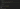In this example, the original array `\$myArray` contains the numbers 1 to 5. The `\$skippedArray` contains all the elements except the first one, which is 1. We used array slicing to create a new array starting from index 1 and ending at the last index of the original array (`\$myArray.Length - 1`).

## Using Select-Object and Skip parameter#

This solution is similar to the previous one, except we use `Select-Object` cmdlet to get array elements from pipeline then using `-Skip` parameter with value `1` to literally skip or exclude the first element.

``````
# Sample array
\$myArray = @(1, 2, 3, 4, 5)

# Skip the first item using Select-Object cmdlet with Skip parameter
\$skippedArray = \$myArray | Select-Object -Skip 1

# Output the result
\$skippedArray

``````

The result will look as follows: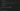## Using Simple Iteration#

In this solution, we use simple iteration to loop through array elements, starting from the second index, which is 1, until the last index (`\$myArray.Length - 1`).

``````
# Sample array
\$myArray = @(1, 2, 3, 4, 5)

# Initialize an empty array to store the result
\$skippedArray = @()

# Use a for loop to iterate over the array starting from index 1
for (\$i = 1; \$i -lt \$myArray.Length; \$i++) {
\$skippedArray += \$myArray[\$i]
}

# Output the result
\$skippedArray

``````

The result will look as follows: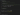## Using ArrayList#

We can also convert the array to `ArrayList` and use `GetRange` method to slice the array.

``````
# Sample array
\$myArray = @(1, 2, 3, 4, 5)

# Skip the first item using GetRange method of ArrayList
\$skippedArray = [System.Collections.ArrayList]::new(\$myArray).GetRange(1, \$myArray.Length - 1)

# Output the result
\$skippedArray

``````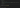## Using LINQ#

We can also use C# LINQ to skip the first element by using `Skip` method. To make it run, we must explicitly cast the first `Skip` method parameter to array of integers because the actual `Skip` method is generic so we must specify the type.

``````
# Sample array
\$myArray = @(1, 2, 3, 4, 5)

# Skip the first item using the Skip method of IEnumerable
\$skippedArray = [Linq.Enumerable]::Skip([int[]]\$myArray, 1)

# Output the result
\$skippedArray

``````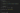## Using PowerShell Ability to Assign Multiple Variables#

We can also make use of PowerShell ability to assign multiple variables. In this case, we assign `\$myArray` to two variables where the first one will hold only the first item and the second one will hold the remaining items.

``````
# Sample array
\$myArray = @(1, 2, 3, 4, 5)

# Assign \$myArray to two variables where the first one will hold only the first item
# while the second one will hold the remaining items
\$first, \$rest = \$myArray

# Output the result
\$rest

``````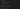## Conclusion#

To skip the first item in an Array, we can use some techniques like array slicing, using combination of `Select-Object` and `Skip` parameter. We can also use simple iteration where we skip the iteration of the first index.

We can also cast teh array to `ArrayList` then get the specific ranges we want. Other than that, we can also use `LINQ Skip` method.

Last, we can make use of PowerShell ability to assign multiple variables. In this approach, we assign the array to two variables where the first one holds the first element and the second one holds the remaining elements.